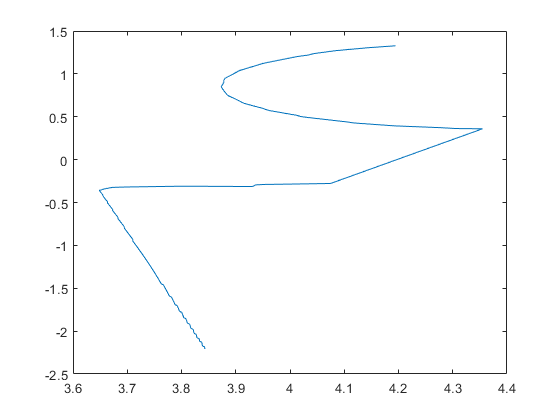Read laser scan ranges in Cartesian coordinates

## Syntax

``cart = readCartesian(scan)``
``cart = readCartesian(___,Name,Value)``
``````[cart,angles] = readCartesian(___)``````

## Description

example

````cart = readCartesian(scan)` converts the polar measurements of the laser scan object, `scan`, into Cartesian coordinates, `cart`. This function uses the metadata in the message, such as angular resolution and opening angle of the laser scanner, to perform the conversion. Invalid range readings, usually represented as `NaN`, are ignored in this conversion.```

example

````cart = readCartesian(___,Name,Value)` provides additional options specified by one or more `Name,Value` pair arguments. Name must appear inside single quotes `('')`. You can specify several name-value pair arguments in any order as `Name1,Value1,...,NameN,ValueN`.```
``````[cart,angles] = readCartesian(___)``` returns the scan angles, `angles`, that are associated with each Cartesian coordinate. Angles are measured counterclockwise around the positive z-axis, with the zero angle along the x-axis. The `angles` is returned in radians and wrapped to the [ –`pi`, `pi`] interval.```

## Examples

collapse all

Connect to ROS network. Subscribe to a laser scan topic, and receive a message.

`rosinit('192.168.17.129')`
```Initializing global node /matlab_global_node_40737 with NodeURI http://192.168.17.1:56343/ ```
```sub = rossubscriber('/scan'); scan = receive(sub);```

Read the Cartesian points from the laser scan. Plot the laser scan.

```cart = readCartesian(scan); plot(cart(:,1),cart(:,2))```Shutdown ROS network.

`rosshutdown`
```Shutting down global node /matlab_global_node_40737 with NodeURI http://192.168.17.1:56343/ ```

Connect to ROS network. Subscribe to a laser scan topic, and receive a message.

`rosinit('192.168.17.129')`
```Initializing global node /matlab_global_node_12735 with NodeURI http://192.168.17.1:56572/ ```
```sub = rossubscriber('/scan'); scan = receive(sub);```

Read the Cartesian points from the laser scan with specified range limits. Plot the laser scan.

```cart = readCartesian(scan,'RangeLimit',[0.5 6]); plot(cart(:,1),cart(:,2))```Shutdown ROS network.

`rosshutdown`
```Shutting down global node /matlab_global_node_12735 with NodeURI http://192.168.17.1:56572/ ```

## Input Arguments

collapse all

`'sensor_msgs/LaserScan'` ROS message, specified as a `LaserScan` object handle.

### Name-Value Pair Arguments

Specify optional comma-separated pairs of `Name,Value` arguments. `Name` is the argument name and `Value` is the corresponding value. `Name` must appear inside quotes. You can specify several name and value pair arguments in any order as `Name1,Value1,...,NameN,ValueN`.

Example: `'RangeLimits',[-2 2]`

Minimum and maximum range for a scan in meters, specified as a 2-element ```[min max]``` vector. All ranges smaller than `min` or larger than `max` are ignored during the conversion to Cartesian coordinates.

## Output Arguments

collapse all

Cartesian coordinates of laser scan, returned as an n-by-2 matrix in meters.

Scan angles for laser scan data, returned as an n-by-1 matrix in radians. Angles are measured counterclockwise around the positive z-axis, with the zero angle along the x-axis. The `angles` is returned in radians and wrapped to the [ –`pi`, `pi`] interval.